Thursday, August 29, 2013

R1.1: Probability Distributions - Random Sampling, Combinatorics in R

In this post we will work with R to perform some statistical tests and sampling using probability distributions.

Not all data sets have exact distributions that match a specific probability density function. There is a certain degree of randomness and 'un-reproducibility' to it. For example, ask 100 people with iPads how many times they use their iPads to view their Twitter feeds, and we will get a set of seemingly random numbers. That is to say, the data is almost guaranteed not to fit a perfect statistical model, such as a normal distribution or a Poisson distribution. However, these theoretical statistical distributions can enable us to have a better understanding and view of the data we work with. Let us dive in!

1. Random Sampling

In R we can draw random samples from a specific distribution. A basic example would be picking numbers for the Powerball lottery. To get the jackpot, we would have to match all six numbers on our \$2 ticket, with five of them ranging from 1 to 59, and the last red ball ranging from 1 to 35 (they are drawn from two separate sets of balls). Here is the drawing of the 'staggering' \$217.2 million Powerball jackpot in February 2013:

The Powerball site gives the odds of winning the jackpot at dismal 1 in 175,223,510 (I do not like it either, but hey, a ticket is only two dollars). We will derive this number further into the post.

To simulate this in R, we can choose sample numbers. The code for the five white balls is followed by the code for the red powerball, with results shown.Fig. 1: Powerball Number Sampling
The the sample function picks a specified number of samples (5 and 1) from a range of numbers (1 to 59 and 1 to 35). We can concatenate the two sets of numbers together with the concatenate function and assign the result to pbdrawing.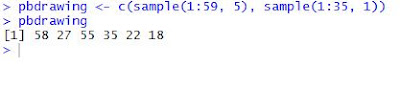Fig. 2: All Six Numbers in a Sample Ticket
You might notice that the numbers 'drawn' the second time in Figure 2 were different than in Figure 1. This is because every time we use the sample function, we get a new set of sampled numbers. However, since we stored them into pbdrawing, the numbers in there will stay the same (unless we assign different numbers to it after).

The default for the sample function is not to replace the values selected. The Powerball numbers are not replaced in the actual drawing. We can tell R to replace by adding a third argument, replace=True. This would be applicable in coin tossing. Say we toss a coin 10 times, assuming equal probability. Just because we toss a heads does not mean we cannot obtain that result again. That is when we set replace to True.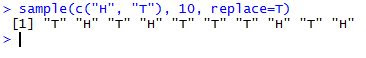Fig. 3: Results of Ten Coin Tosses
As you can see, we get 6 tails and 4 heads from 10 tosses. The result is not split down the middle, because it is random  sampling. If we would like to split the probability unevenly, we can alter the probability of the events in the sample range we are picking from. Suppose we have an electronic prototype, say the next generation iPads, which has a 10% screen failure rate after a month of testing (ouch, glad it is still a prototype). We can take a random sample of 10 next-gen iPads and see how many screens failed at the end of the testing period.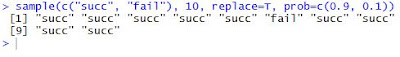Fig. 4: Successes and Failures
We represented the good iPads by "succ", short for success, and the iPads with bad screens with "fail". The additional code redefines the probability to be 0.9 for the first outcome (good screen!), and 0.1 (or 10%) for the second outcome of screen failure. So we have 9 iPads with good screens and 1 with a bad screen, which is about what we could expect in a random sample of 10 iPads.

2. Probability Calculations & Combinatorics

Let us go back to the Powerball example using combinatorics. To recap, Powerball drawings occur from drawing 5 numbers from balls labeled 1 through 59 and one red ball from balls labeled 1 through 35.

If we draw the first five white balls, how many different permutations would be possible? That is, how many different ways can we draw the 5 numbers out of the 59? Suppose we take into account the order of the numbers. Then we would calculate the permutation using factorials (where 4! is 4*3*2*1).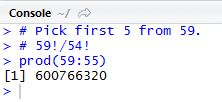Fig. 5: Permutation of Picking 5 from 59 numbers
The number of ways we can pick from 59 white balls is 59, and after the first pick, we have 58 left (no replacement). And choosing from the 58 there are 58 different balls, etc. So we need 59*58*57*56*55. We can write it using factorials: 59!/54! works because the numbers 54 and below in the denominator cancel out the numbers 54 and below in the numerator. Therefore we are left with: the product of numbers 59 to 55, as prod(59:55)as shown in Figure 5. R tells us that there are 600,766,320 ways (in order) we can pick 5 numbers from 59 balls.

But in the Powerball lottery, it does not matter what order the numbers are picked (like in the YouTube video), you just need to match them. So order does not matter; and to calculate how many ways we can choose 5 numbers from 59 balls in any order, we need to understand combinations.

We start with the permutation we calculated previously. That large number (6 hundred million+), is the number of ways we can choose 5 numbers in unique order. If order does not matter, the number of ways will be less. So we can simply divide it with the number of ways the 5 chosen numbers can be ordered. The first lucky number has 5 locations, 1st, 2nd, 3rd, 4th, or 5th one chosen, and the second number has 4 locations, etc. Therefore, we take 5! for the number of balls we picked, and use it to divide the permutation.Fig. 6: Combination of White Powerball Numbers
In Figure 6, we see the permutation, followed by the combination calculation, and then by the handy R function for combination, the choose function. We observe the same number of ways for the choose function and our manual calculation derived from permutation.

The mathematical formula for combination (also known as the binomial coefficient), where n is the number of elements and k is the number of picks: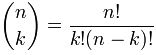Fig. 7: Number of k-Combinations in n-Elements
and is commonly read as "n choose k" (hence the name of the R function).

So when choose the final red ball from 35 balls, there is 35 different balls to choose from. We can apply the formula (however obvious the solution) to verify: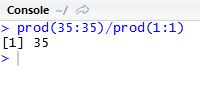Fig. 8: Combinations of the Red Ball
Needless to say, the 34! cancels out all but the 35 in the 35! and 1! is simply 1, so the result is 35 different ways to choose a red ball from 35 of them. Now with different sets of balls, multiplying the number of ways to choose the white ones and the red one will give us the number of possible ways the Powerball lottery can draw its numbers.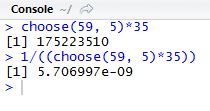Fig. 9: Combination of White Balls and Red Ball with Probability
As we can see, the result of 175,223,510 is the number of different combinations of Powerball ticket numbers. This matches the odds of winning the jackpot, as given on their website. The probability, frankly, does not look good. At all. It looks like we have a 0.000000571% chance of winning the jackpot with any random ticket (that is a lot of zeros).

However you have to play for a chance of winning (a ticket to dream, in my opinion). So best of luck when playing Powerball!

In the next post for Probability Distributions in R, we will cover calculations from different probability distributions.

1.